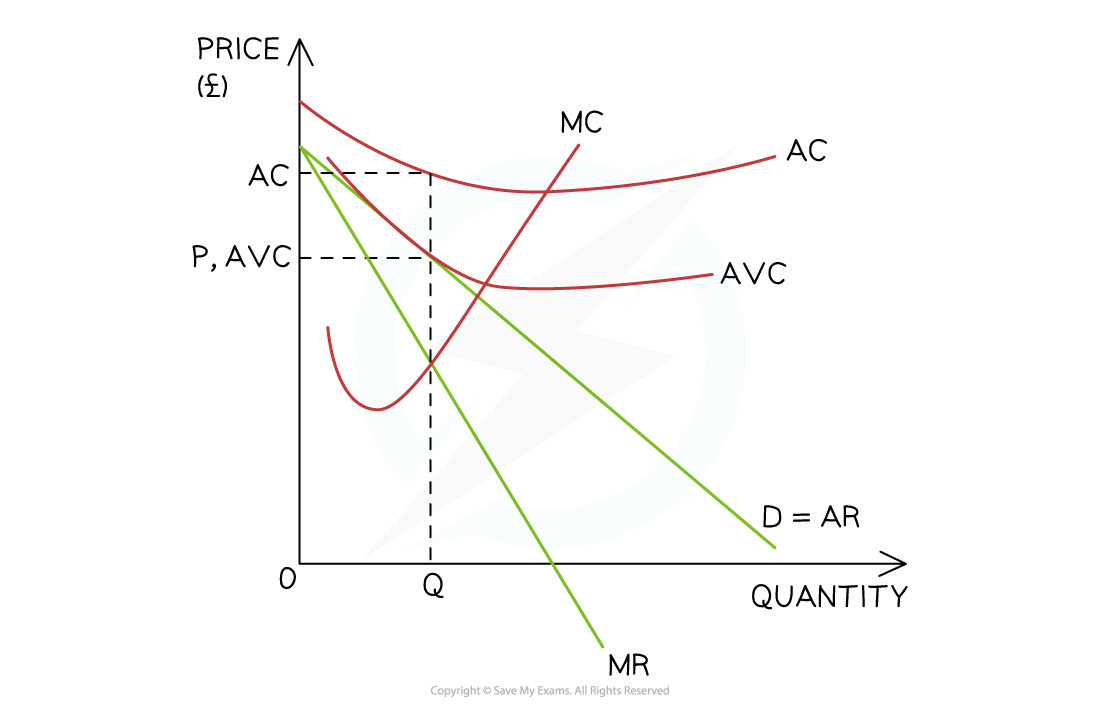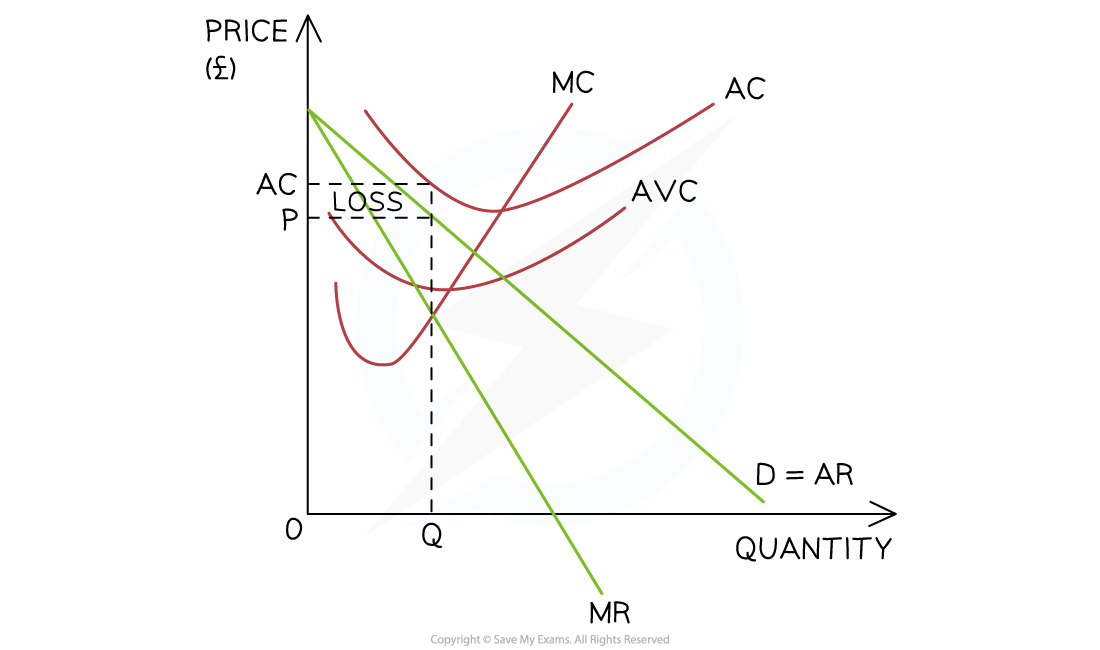# Edexcel A Level Economics A:复习笔记3.3.4 Normal Profits, Supernormal Profits & Losses

### Condition for Profit Maximisation

• To maximise profit firms should produce up to the level of output where marginal cost (MC) = marginal revenue (MR)

Calculations To Demonstrate the Profit Maximisation Rule

 Output MR (£) MC (£) Addition to Profit 5 50 32 +18 6 50 36 +14 7 50 50 0 8 50 68 -18

Observations

• With the 7th unit of output, MC = MR & no additional profit can be extracted by producing another unit
• Up to the 6th unit of output, MC < MR & additional profit can still be extracted by producing an additional unit
• From the 8th unit of output, MC > MR & the firm has gone beyond the profit maximisation level of output
• It is making a marginal loss on each unit produced beyond the point where MC = MR

### Normal Profit, Supernormal Profit & Losses

• When calculating costs, Economists consider both the explicit and implicit costs of production
• Explicit costs are the costs which have to be paid e.g raw materials, wages etc.
• Implicit costs are the opportunity costs of production
• This is the cost of the next best alternative to employing the firm's resources
• E.g. if an investor puts £1m into producing bicycles & they could have put it in the bank to receive 5% interest, then the 5% represents an implicit cost
• Implicit costs must be considered as entrepreneurs will rationally reallocate resources when greater profits can be made elsewhere
• Profit = total revenue (TR) - total costs (TC)
• Total costs include explicit and implicit costs
• Normal profit occurs when TR = TC
• This is also called breakeven
• Supernormal profit occurs when TR > TC
• A loss occurs when TR < TC

Calculations To Demonstrate Profits

 Output TR (£) TC (£) Profit (TR - TC) 5 150 70 80 6 180 96 84 7 220 220 0 8 250 270 -20

Observations

• Supernormal profit occurs up to the 6th unit of output
• Normal profits occur at the 7th unit
• From the 8th unit, the firm is making a loss

### Short-run & Long-run Shut-down Points

• Firms do not always make a profit & may endure losses for a period
• Entrepreneurs often keep firms going in the hope that market conditions will change & demand for their products will increase leading to profitability
• This raises the question, 'when is it the best time for a firm to shut down?'
• The shut-down rule provides the answer by considering both the long-run & short-run periods

#### The Short-run Shut Down Point

• In the short-run, if the selling price (average revenue) is higher than the average variable cost (AVC), the firm should keep producing (AR > AVC)
• If the selling price (AR) falls to the AVC it should shut down (AR = AVC)A firm should shut down in the short-run if the selling price (AR) is unable to cover the AVC

Diagram analysis

• The firm produces at the profit maximisation level of output (Q) where MC=MR
• At this level, the P = AVC
• This means that there is no contribution towards the firm's fixed costs
• The selling price literally only covers the cost of the raw materials used in production
• There is no point in continuing production & the firm should shut down

#### The Long-run Shut Down Point

• In the long-run, if the selling price (AR) is higher than the average cost (AC) the firm should remain open (AR > AC)
• if the selling price (AR) is equal to or lower than the average cost (AC), the firm should shut down (AR = AC)A firm should shut down in the long-run if the selling price (AR) is unable to cover the AC

Diagram analysis

• The firm produces at the profit maximisation level of output (Q) where MC=MR
• At this level, P < AC
• It could continue operating in the short-run as the AR > AVC, but in the long-run they are making a loss & the firm will shut down